# Dissolving the Pentagram

A week ago Denis asked the GGL-list if the internal segments of a self-intersecting polygon could be dropped. He attached this figure:I answered that dissolve should do this. But it does not in this case. Dissolve can remove self-intersections, but under certain circumstances and even then it is not always perfect. So this is a reason to remove dissolve to the extensions folder - it should not yet be part of Boost.Geometry as it is released, hopefully in Boost 1.47

## Pentagram

A pentagram is a five pointed star which can be drawn with a linestring of five segments. This is a Well-Known text presentation of a pentagram:

POLYGON((5 0,2.5 9,9.5 3.5,0.5 3.5,7.5 9,5 0))

As I often do, I compare the behaviour of algorithms on this WKT with SQL Server, PostGIS, and other packages (in this case Quantum GIS and SVG).

## SVG

Boost.Geometry can create SVG images. This is really useful and I often use it in test programs. SVG's can then be depicted in FireFox. (In Google Chrome dropping the second SVG let it crash...).

SVG has thousands of properties, and one of them is the fill-rule. It can be set to winding and to non-zero. This delivers two different presentations: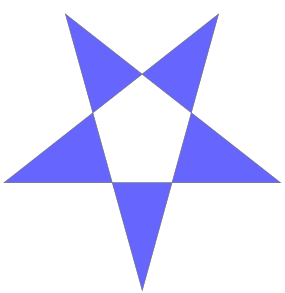winding non-zero

## SQL Server

In SQL Server the pentagram is drawn as such: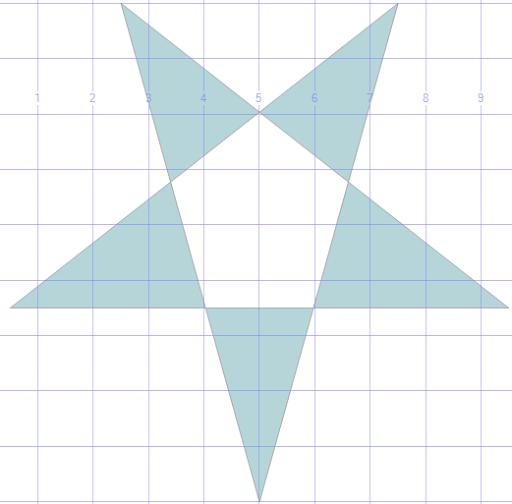So it is using the winding rule.

The polygon is not valid, of course, it has five self-intersection points. SQL Server has a very good method to make polygons valid. It is obviously called MakeValid(). It is a SQL Server extension, not an OGC Method. I wrote "very good" and I mean that, we used it a lot in real-world problems and it has always worked very well.

With this specific pentagram MakeValid() creates a multi-polygon containing five triangles. So it directly uses the winding rule to create a multi-geometry. Sounds logical. If we calculate the area we get 17.7316840044235, the summed area of the five triangles. Calculating the area of a non-valid polygon is not possible in SQL Server. The statement is:
```
select geometry::STGeomFromText('POLYGON((5 0,2.5 9,9.5 3.5,0.5 3.5,7.5 9,5 0))',0)
.MakeValid().STArea()
```

## PostGIS

PostGIS does not have a drawing engine. In PostGIS we can calculate the area of an invalid polygon and it gives us: 33.5. There is (AFAIK) not yet a function to make polygons valid, though it is announced. The good old trick is to use a buffer with buffer-distance 0, so we do this:

`select ST_Area(ST_Buffer(ST_GeomFromText('POLYGON((5 0,2.5 9,9.5 3.5,0.5 3.5,7.5 9,5 0))',0),0))`
and we get an area of 25.6158419936921. If we draw the zero-buffered result we get a filled polygon.

## Boost.Geometry

Boost.Geometry can calculate the area of an invalid polygon and it also gives us: 33.5. The simple always-working Surveyor's formula just calculates this for us.

If we use the algorithm dissolve we indeed get a polygon with all internal corners dropped in this case. The area of the dissolved polygon is 25.6158412 and this is the right area. So the Surveyor's formula actually cannot be used with invalid polygons, SQL Server is right to prohibit this.

The SVG files displayed above are both created using Boost.Geometry so no picture here.

## Quantum GIS

Quantum GIS has a great extension, QuickWKT, with which WKT geometries can be drawn. It uses the winding rule. It can calculate the area of the polygons (using the Info tool we can get it) and we get 33.5. Right, the Surveyor's formula.## What should happen

What should happen is not that simple. In these four programs, only SQL Server was consistent by prohibiting area calculation and creating a multi-geometry which looks like the image it depicts. All other programs and libraries were consistent, using the Surveyor's formula and creating a closed five-pointed polygon.

The reasoning of Boost.Geometry is that it takes direction into account. So if we draw arrows aside of all linestrings, we get: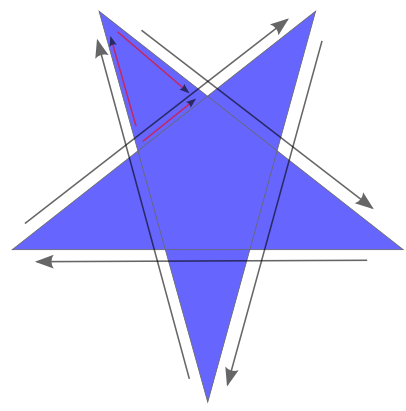And here we see, in the triangle with the smaller arrows, that the direction is not consistent. That triangle should not be generated. Of this whole pentagram several polygons can be drawn with consistent directions, and the largest of them is the whole polygon. So the dissolve function is expected to generate a five-pointed star here. And it does.

There is also a pentagon in the center with consistent directions. Because it has the same orientation as the outer polygon, it should be discarded. And it is. If it would have been orientated counterclockwise, a hole should have been formed.

So there are reasons to say that this pentagram indeed should be converted into a solid five-pointed star. There is no winding rule or non-zero rule involved there, it is just derived by the directions of the segments created by the intersection.

## But now the sample

Anyway, using the sample of Denis Pesotsky, Boost.Geometry's results were not that clever. It generates two polygons which are indeed as expected, with consistent directions. The outer polygon does not have a consistent direction and therefore should not be generated, correct. But there is one polygon with consistent direction missing, in the lower right. That one is discarded but should not have been...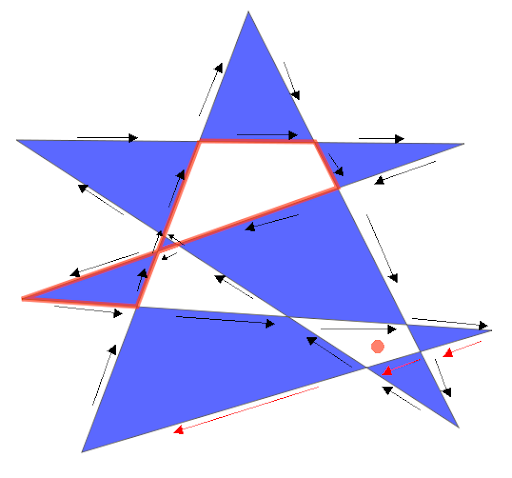Because this, and because we have to think more about the behaviour, the dissolve algorithm has to be moved to the extensions folder. That means it will not be part of the initial release of Boost.Geometry, in the Release branch.

This is the WKT of the input polygon:

POLYGON((55 10, 141 237, 249 23, 21 171, 252 169, 24 89, 266 73, 55 10))

The white pieces are by the winding rule, and in SQL Server it is looking the same (no picture here). SQL Server's MakeValid() creates 9 polygons here.

PostGIS, using the buffer, creates one polygon, looking like this:And that polygon is indeed the largest polygon which can be formed, following all arrows in clockwise direction. So this is the polygon that was expected by the dissolve algorithm...

Anyway, it is not the result that Denis asked for.

## Useful usage

Dissolve can be very useful so I end this blog by just listing some of the testcases where it makes sense:

Inaccurate corners (extra intersection):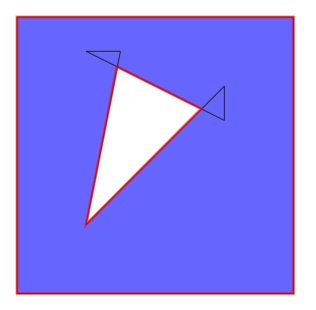Messy corners: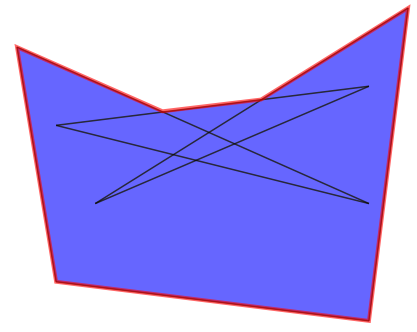Keyholed holes: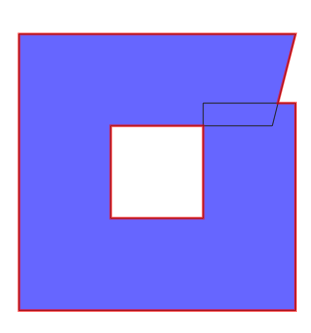## Conclusions

Due to shortcomings in the dissolve algorithm and vagueness in behaviour in general we have to move the dissolve function to the extensions folder. It has to be thought over more thoroughly.